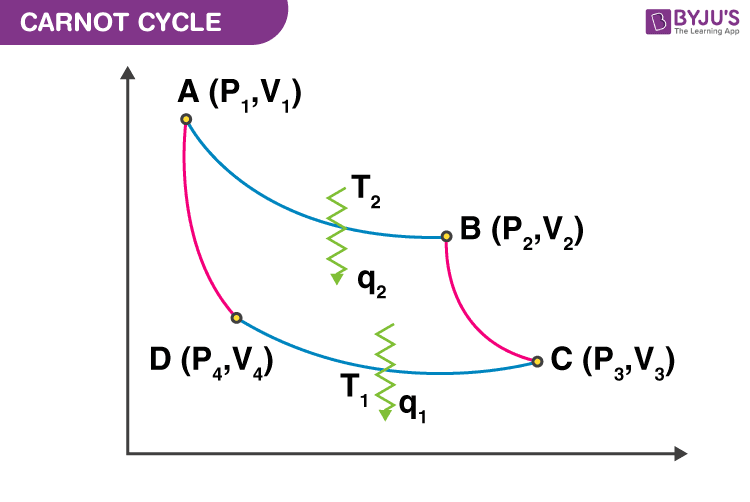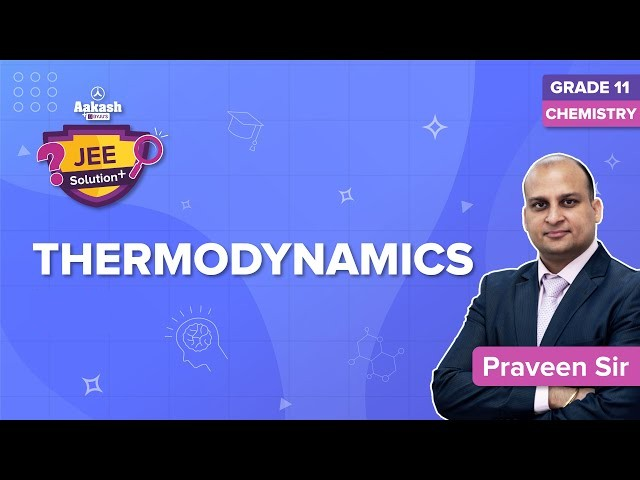Know more about the Repeaters Batch Know more about the Repeaters Batch

# Thermodynamics IIT JEE Study Material

Thermodynamics deals with the interaction of one body with another in terms of quantities of heat and work. In thermodynamics, the system is defined as a quantity of matter or a region in space under investigation. Everything external to the system is the environment or surroundings. The imaginary or actual surface separating the system from its surroundings is known as the boundary. It may be either fixed or movable.

## Closed system (m = constant):

When there is an exchange of energy (work and heat) but not matter between the system and surrounding the system is known as control mass or closed system. For Example, a greenhouse is a closed system that exchanges heat but not work with its surroundings. Depending on the properties of its boundary a system exchanges work, heat, or both. An Adiabatic boundary does not allow the exchange of heat from the system to surroundings and a rigid body does not allow the exchange of work between system and surroundings.

## Isolated systems:

An isolated system does not allow the exchange of heat, work, or matter between the system & surroundings. For example, an insulated container, such as an insulated gas cylinder.

i.e min=mout=Ein=Eout=0

## Open system:

In an open or control volume system, there is an exchange of energy i.e. heat, work, and matter between the system & surroundings. A boundary that allows the exchange of matter is known as the permeable boundary. For example, the ocean, turbine, water heater, car radiator, nozzle, etc. To analyze an open system, the energy of the system is always taken equal to the energy leaving the system.

Other Type of Systems:

Rigid system: It is a type of closed system in which the system interacts with the surroundings by exchanging heat only.

Adiabatic system: It is a closed or open system in which energy is transferred to its surroundings only as work (without transfer of heat or matter).

## Properties of a System:

Intensive properties: Intensive properties are independent of the size of a system. For example, Density, Molar volume, Surface tension, Refractive index, Viscosity, Specific heat, temperature, Free energy per mole, pressure, etc. They are not additive in nature.

Extensive properties: These are properties that are dependent on the size of the system. For example, the number of moles, volume, mass, entropy, free energy, enthalpy, heat capacity, internal energy, etc. They are additive in nature. The specific properties are per unit mass extensive properties. For example, specific mass, specific energy, specific volume, etc.

## Thermodynamic Process:

The Thermodynamic process deals with the change of a system’s state from one state to another. The different types of thermodynamic process are Isothermal, Adiabatic, isobaric and isochoric.

In an Isothermal process, the temperature of the system remains constant [dE and dT = 0] during the state change. This process is carried out in a thermostat and the energy exchange takes place without any change in temperature. In an Adiabatic process, the exchange of energy between the system and surroundings does not occur [dQ = 0]. It is carried out in a perfectly insulated container. The temperature of the system might change during an adiabatic process. In an Isobaric process, the pressure of the system remains constant [dP = 0]. In an Isochoric process, the volume of the system remains constant [dV = 0].

Cyclic Thermodynamic Process [ΔH = 0 and ΔE = 0]:

1. In a cyclic process, a system returns to its initial state after undergoing a number of different thermodynamic processes.

2. A process that is carried out on its own without any external help is known as the spontaneous process.

3. In the Quasi-Static or reversible process, the system and its surroundings can be returned from its final state to its initial state without any changes in the thermodynamic properties of the universe. The irreversible processes are also known as the natural processes because all the processes that occur in nature are irreversible processes. It occurs due to the finite gradient between the two states of the system.

## Zeroth law of Thermodynamics:

According to the Zeroth law of Thermodynamics if two thermodynamic systems are in thermal equilibrium with the 3rd system then all the three systems are in thermal equilibrium with each other.

## First Law of Thermodynamics:

ΔE = Q + W

Where,

ΔE = change in the internal energy of system

Q = Heat transfer (to or from the system)

W = work done (either by the system or on the system)

If a system expands at a constant pressure P from volume V1 to V2, then the 1st law equation can be rewritten as,

ΔE = q – P ΔV [ΔV= V2 -V1]

Work done in different Thermodynamics Process:

In an irreversible process, Work Done

$$\begin{array}{l}W =\mathbf{-\;P_{Ext}\;\;\Delta V}\end{array}$$
[Expansion]

In an isothermal reversible process, Work Done

$$\begin{array}{l}W =\mathbf{-\;2.303\;n\;R\;T\;\;log\;\;\frac{V_{2}}{V_{1}}}\end{array}$$

Also,

$$\begin{array}{l}W = \mathbf{-\;2.303\;n\;R\;T\;\;log\;\;\frac{P_{1}}{P_{2}}}\end{array}$$

In an Adiabatic reversible process, Work Done

$$\begin{array}{l}W = \mathbf{\frac{n\;R}{\gamma \;-\;1}\;\;[T_{2}\;-\;T_{1}]}\end{array}$$

Where, γ = Poisson’s Ratio

$$\begin{array}{l}=\mathbf{\frac{C_{P}}{C_{V}}}\end{array}$$

## Entropy (S):

Entropy changes with the change in the state of a system. The entropy change is given by:

$$\begin{array}{l}\mathbf{\Delta \;S\;=\;\frac{q_{rev}}{T}}\end{array}$$

Where qrev = heat supplied reversibly and isothermally.

Entropy is maximum when the system is in equilibrium i.e.

$$\begin{array}{l}\mathbf{\Delta \;S\;=\;0}\end{array}$$

## Second Law of Thermodynamics:

The Second Law of Thermodynamics states that the state of the entropy of the entire universe is always increasing with time. Also, the changes in the entropy in the universe can never be -ve.

## Carnot Cycle:Form the above Carnot cycle PV diagram:

1. A → B is an isothermal reversible expansion process

2. B → C is an adiabatic reversible expansion process

3. C → D is an isothermal reversible compression process

4. D → A is an adiabatic reversible compression process

Now, the work done in different thermodynamic processes:

• $$\begin{array}{l}\mathbf{W_{AB}\;=\;-\;n\;R\;T_{2}\;ln\;\frac{V_{2}}{V_{1}}}\end{array}$$
• $$\begin{array}{l}\mathbf{W_{BC}\;=\;C_{v}\;(T_{1}\;-\;T_{2})}\end{array}$$
• $$\begin{array}{l}\mathbf{W_{CD}\;=\;-\;n\;R\;T_{1}\;ln\;\frac{V_{4}}{V_{3}}}\end{array}$$
• $$\begin{array}{l}\mathbf{W_{DA}\;=\;C_{v}\;(T_{2}\;-\;T_{1})}\end{array}$$

Carnot Efficiency:

$$\begin{array}{l}\mathbf{eta \;=\;-\;\frac{W_{Total}}{q_{2}}\;=\;\frac{T_{1}\;-\;T_{2}}{T_{2}}\;=\;\frac{q_{1}\; +\;q_{2}}{q_{2}}}\end{array}$$

## Entropy Change:

$$\begin{array}{l}\mathbf{\Delta \;S\;=\;n\;C_{v}\;ln\;\frac{T_{2}}{T_{1}}\;+\;n\;R\;ln\;\frac{V_{2}}{V_{1}}}\end{array}$$

1. Reversible irreversible isothermal contraction and expansion: (ideal gas)

$$\begin{array}{l}\mathbf{\Delta \;E\;=\;0,\;\Delta \;H\;=\;0, \;and\;\Delta \;S\;=\;n\;R\;ln\;\frac{V_{2}}{V_{1}}}\end{array}$$

2. Isobaric Heating or Cooling:

$$\begin{array}{l}\mathbf{\Delta \;E\;=\;C_{v}\;\Delta T,\;\Delta \;H\;=\;q_{p}\;=\;C_{p}\;\Delta T, \;and\;\Delta \;S\;=\;n\;C_{p}\;ln\;\frac{T_{2}}{T_{1}}}\end{array}$$

3. Isochoric Heating or Cooling:

$$\begin{array}{l}\mathbf{\Delta \;E\;=\;q_{v}\;=\;C_{v}\;\Delta T,\;\Delta \;H\;=\;C_{p}\;\Delta T, \;and\;\Delta \;S\;=\;n\;C_{v}\;ln\;\frac{T_{2}}{T_{1}}}\end{array}$$

$$\begin{array}{l}\mathbf{\Delta \;E\;=\;C_{v}\;\Delta T,\;\Delta \;H\;=\;C_{p}\;\Delta T}\end{array}$$

5. For Irreversible process:

$$\begin{array}{l}\mathbf{\Delta \;S\;=\;n\;C_{v}\;\ln\;\frac{T_{2}}{T_{1}}\;+\;n\;R\;ln\;\frac{V_{2}}{V_{1}}}\end{array}$$

6. For Reversible adiabatic compression and expansion:

$$\begin{array}{l}\mathbf{\Delta \;S\;=\;0}\end{array}$$

## Third law of Thermodynamics:

Statement: At absolute 0 Temperature, the entropy of a perfectly crystalline substance is 0.

By utilizing the third law of thermodynamics, the absolute value of entropy can be evaluated at room temperature (for pure substances). The standard absolute entropy is the entropy of a substance in its standard temperature and pressure i.e. at 1 atm and 298 K.

The absolute entropies can be utilized in calculating the changes in entropy by using the relation:

$$\begin{array}{l}\mathbf{\Delta \;S^{\circ}\;=\;\sum \;\;S^{\circ}\;\;(Products)\;-\;\sum \;S^{\circ}\;\;(Reactants)}\end{array}$$

## Gibbs Free Energy:

G = H – TS and at constant pressure and temperature

$$\begin{array}{l}\mathbf{\Delta \;G\;=\;\Delta \;H\;-\;T\;\Delta \;S}\end{array}$$

1. For Reversible process:

$$\begin{array}{l}\mathbf{\Delta \;G\;\leq \;0}\end{array}$$

2. For Spontaneous Process:

$$\begin{array}{l}\mathbf{\Delta \;G\;< \;0}\end{array}$$

3. For Equilibrium:

$$\begin{array}{l}\mathbf{\Delta \;G\;=\;0}\end{array}$$

### Thermodynamics Important Topics### Thermodynamics Important Questions### Thermodynamics Top 7 Questions### Thermodynamics JEE Solutions### Thermodynamics JEE Main Important Questions### Top 15 Most Important and Expected JEE Questions of Thermodynamics## Thermodynamics – Most Important JEE Advanced Questions## Frequently Asked Questions – FAQs

### What is the equation of the first law of thermodynamics?

ΔU = ΔW – ΔQ is the equation of the first law of thermodynamics

### What are intensive properties?

A property that is independent of the mass of a substance is called an intensive property. For example, Temperature is an intensive property.

### What are extensive properties?

The property that is dependent on the mass of a substance is called an extensive property. For example, volume is an extensive property.

### Is density an intensive property or extensive?

The ratio of two extensive properties is always intensive. Density is the ratio of two extensive properties- mass and volume. Thus density is an intensive property.

### What is the meaning of entropy of a system?

The randomness of a system is called the entropy of the system. The entropy of the universe is always increasing.
Test your knowledge on Thermodynamics For Iit Jee